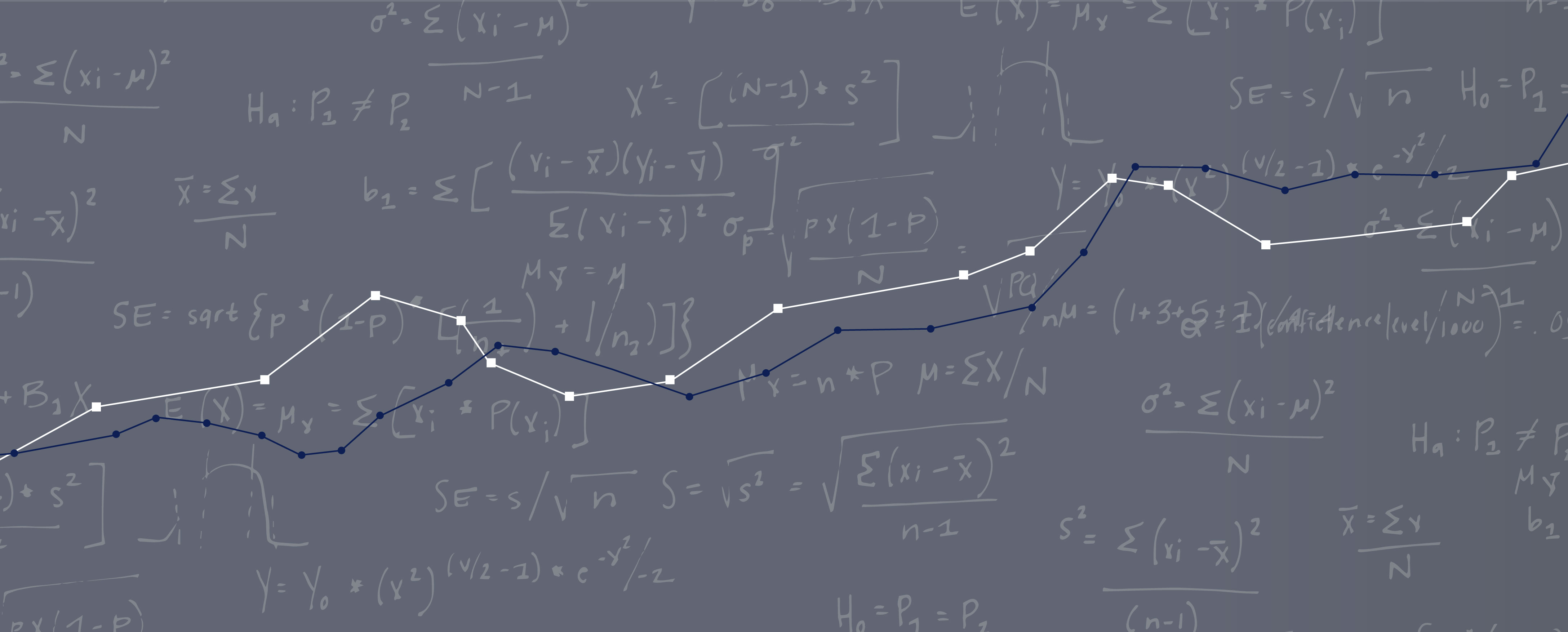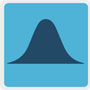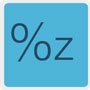# Calculators#### A/B Test Calculator#### Confidence Interval Calculator#### Compare 2 Small Sample Completion Rates (Fisher Exact Test)#### One Sample Proportion Calculator#### Sample Size Calculator for a Completion Rate#### Interactive Graph of the Standard Normal Curve#### Percentile to Z-Score Calculator#### Z-Score to Percentile Calculator#### Sample Size Calculator for Discovering Problems in a User Interface#### Graph and Calculator for Confidence Intervals for Task Times#### One Sample Proportion Calculator#### Sample Size Calculator for a Completion Rate#### Sample Size Calculator for Discovering Problems in a User Interface#### A/B Test Calculator#### Compare 2 Small Sample Completion Rates (Fisher Exact Test)#### Percentile to Z-Score Calculator#### Z-Score to Percentile Calculator#### Confidence Interval Calculator#### Confidence Interval Calculator for a Completion Rate#### Interactive Graph of the Standard Normal Curve